# How to Group Dates or A Period by Month/Quarter/Year in Pivot Table?

A pivot table is a table format that can dynamically arrange data and do category summarize strongly. It is commonly used in our daily life for creating summary report. It provides various combinations for row/column/values. Normally we can get the proper table format as we expect after setting group for these fields. As date or a period is an important parameter in summary report, in this article, we will introduce you how to group date (or a period of dates) by month/quarter/year in pivot table. This will help you to arrange your table group by different time settings flexible.

Initial Condition:

#1 Prepare a table with Product, Sales and Date.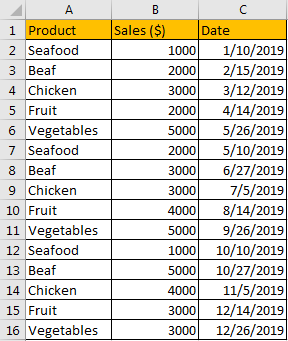#2 Then create pivot table for this table, then we can analysis data conveniently. Select range A1:C16, then click Insert->PivotTable, choose existing worksheet, locate pivot table in E1, then click OK.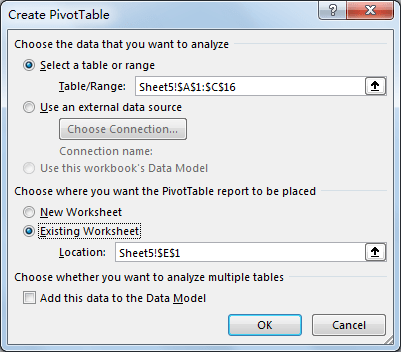#3 Verify that after clicking OK, PivotTable Fields settings pops up. Check on Product, Sales (\$), Date in left control panel, and move Date to the top in Rows.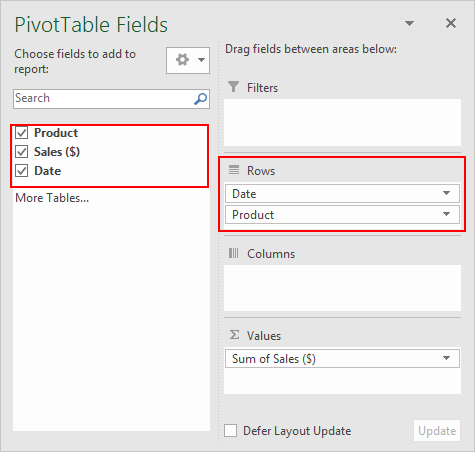#4 Verify that pivot table is created properly. You can find that sales for all products are grouped by Year in this table.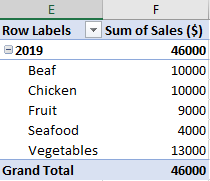## Group Date or A Period by Month/Quarter/Year in Pivot Table

In above case, we know that date is grouped by Year by default. So, we just need to know how can we change Year to Month or others in pivot table.

Step 1: Select Year 2019 in pivot table, then right click to load menu, select Group.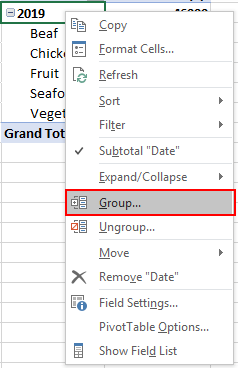Step 2: In Grouping window, uncheck Years (which is selected by default), then select Months, then click OK.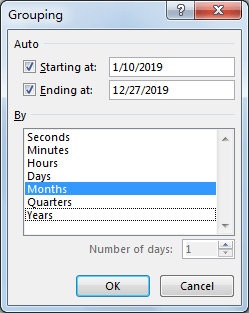Step 3: Verify that date is grouped by Months properly. Due to length limit, only part of table is displayed in below screenshot.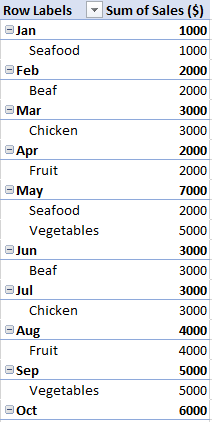Step 4: Repeat step#2, this time you can check on both Year and Month to see the difference.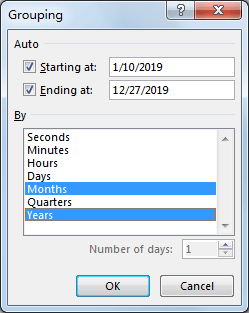Step 5: Click OK on above screenshot. Verify that this time, Year is added properly.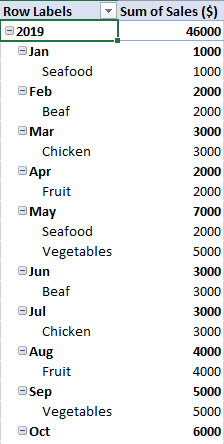You can click ‘-‘ before 2019 to shrink months. You can also click ‘+’ to spread all months.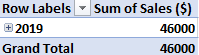Step 6: Repeat step#2 to enter Grouping again. This time select Quarters.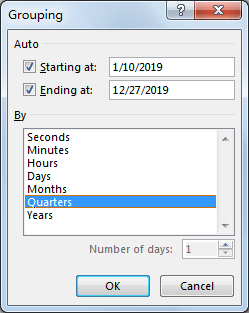Step 7: Click OK. Verify that date is grouped by quarters properly.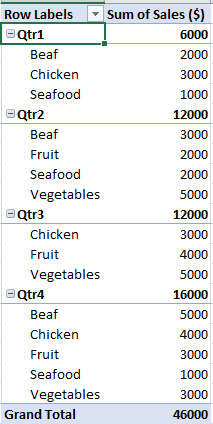Comment:

If you just want to group a period of dates by months/quarters/year and others are not grouped, you can set start date and end date in Grouping. For example, enter 7/10/2019 as Starting at value, 10/10/2019 as Ending at value.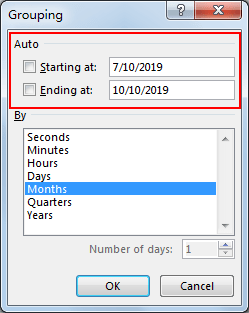Click OK. Verify that only this period is grouped by months.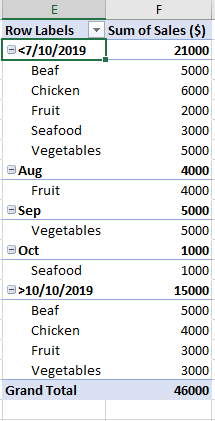Related Posts

Calculate Total Cost with Excel VLOOKUP Function

In today's article we will show you how to calculate the total cost for a given weight using the Excel VLOOKUP function. This function will help us to find the appropriate unit price for that weight and then we can ...

Excel Formulas To Calculate The Bond Valuation

Assume that you've been assigned a task of calculating bond valuation; so if you are new to Ms Excel or do not have much experience with it, then I am pretty sure about it that doing this task manually might ...

Calculate Cumulative Totals with Excel SUM Function

Today, through a simple example, we will show you how to use one of the most common-used Mathematical functions in excel, the SUM function, to add up the sum. In our daily life, we keep an account of what we ...

BMI Calculation Formula In Ms Excel

You might have come across a task in which you were assigned to make BMI calculations of the supplied numbers, and you may be looking for an efficient approach to accomplish this process rather than doing BMI calculations manually, by ...

Calculate The Period of Loan or Investment in Excel

In our daily life, most of us will invest or take a loan, so we need to master some simple financial functions to calculate the period needed for loans or investments. If you work as an accountant, you need to ...

Working Time Calculation Based on Timesheets

In the office, a special machine record the time when you start working (clock in office) and when you finish working (clock out of office). We can calculate the total working time by subtracting the end working time from the ...

Basic Price Discount Calculation with Excel VLOOKUP Function

We often encounter product discounts in our shopping. Depending on the level of spending, the mall will offer different percentages of discounts. Usually, the more you spend, the bigger the discount, while the less you spend, the smaller the discount. ...

Sort/Rank Numeric Values with Duplicate Values Exist

Excel built-in RANK function can sort a set of values. If there are duplicate numbers, then the rank number is also duplicated. See the following example: There are two numbers “100” in range A2:A9, they are both the third largest ...

Calculate Days Open in Excel

If you want to know how to Calculate days in Excel, there are some formulas that you can use to do so. For example, you can use the DAYS function in Excel to find the number of days between two ...

Calculate Grades With VLookup in Excel

Why Should You Calculate Grades With VLookup Excel? If you're looking for a simple way to find out a student's grades, VLOOKUP Excel can do the trick. This function uses a lookup table to find the values and sort them ...

Sidebar